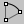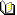Arc

Draws an arc curve.

Steps:

• Follow the prompts for the selected option. If no option is specified, the default option is used.

Options

Default (Center/Angle)

Creates an arc using the arc’s center location and an angle.

1. Pick
the center of the arc’s radius.

2. Pick the start of the arc.

3. Pick the end point of the arc, or, type the angle for the arc.

Tilted

Draws the arc in 3-D space, off the construction plane.

1. Pick
the start that is not on the construction plane.

2. Pick an orientation.

Deformable

Creates an arc-shaped NURBS curve

.

StartPoint

Draws an arc from start point, end point, and a point on the arc.

1. Pick
the start of the arc.

2. Pick the end of the arc.

3. Pick a point along the arc.

Options

Direction

1. Pick
the tangent direction at the start of the arc.

2. Pick the end of the arc.

ThroughPoint

1. Pick
a point along the arc to pass through.

2. Pick the end of the arc.

Tangent

Draw an arc of specified radius tangent to two curves.

1. Drag the indicator and pick
the first tangent curve.

2. Drag the indicator and pick the second tangent curve, or type a radius.

3. Drag the indicator and pick the third tangent curve.

4. At the Choose arc prompt, move your mouse to view the possible arcs and click to select.

Options

Point

Releases the command from the tangent constraint.

FromFirstPoint

Constrains the cursor to the picked location on the first tangent curve.

The arc is restricted to the specified radius. If a tangent point exists on the second curve that meets the radius requirement, the tangent constraint will appear at that point as you drag the arc around.

Extension

Extends a curve with an arc.

1. Select a curve near the end.

2. Pick the end point of the arc.

Option

Center

1. Pick the center of the arc extension.

2. Pick the end point of the arc.

Notes

• To draw an arc extension and join it in one step, use the Extend
command.

• The center point is constrained to a plane perpendicular to the curve end. If the extension is drawn in a view perpendicular to the constraint plane, the input will be automatically locked parallel to the construction plane
.

• In other views Rhino tries to make sure one of the directions is parallel to the construction plane
. Press the Shift

key when making the pick to maintain the ortho direction.Arc > Arc: Center, Start, Angle Main1 > Arc: Center, Start, AngleCurve > Arc > Center, Start, AngleRelated topics…